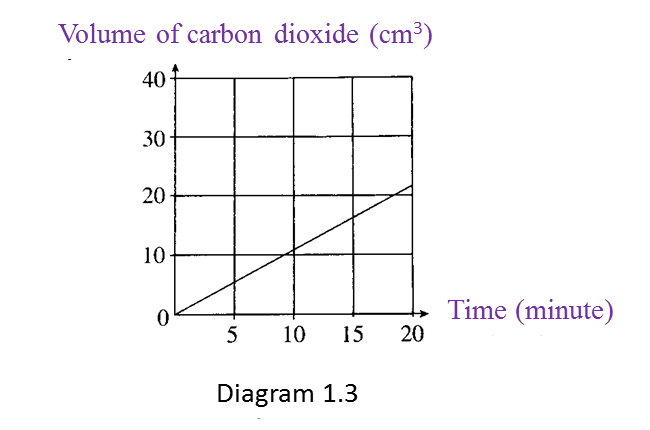Question 1:
Diagram 1.1 and Diagram 1.2 show an experiment to investigate the effect of temperature on the fermentation of glucose by yeast.(a) State one hypothesis that can be made from this experiment. [1 mark]

(b) State the variables in this experiment.
(i) Manipulated variable [1 mark]
(ii) Responding variable [1 mark]

(c) Based on Diagram 1.1 and 1.2, which temperature is more suitable for the fermentation of glucose? [1 mark]

(d) Diagram 1.3 shows the graph of the volume of carbon dioxide produced at 35oC against time.What is the relationship between the volume of carbon dioxide produced and time? [1 mark]